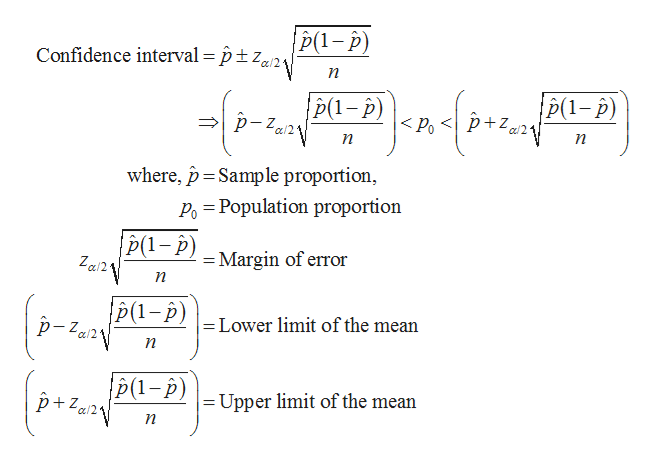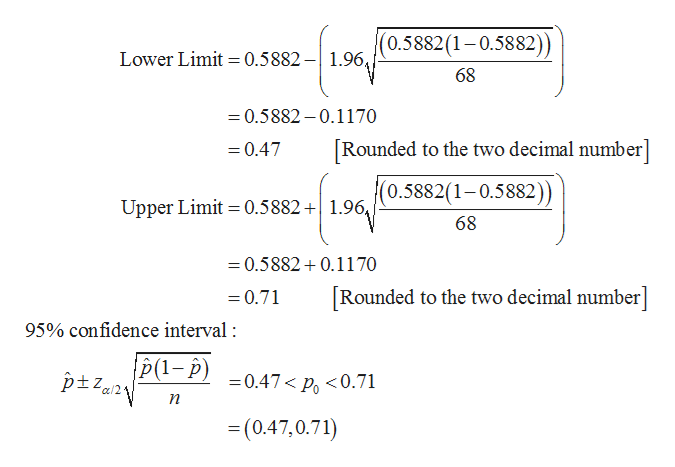# For this problem, carry at least four digits after the decimal in your calculations. Answers may vary slightly due to rounding.In a random sample of 68 professional actors, it was found that 40 were extroverts.(a) Let p represent the proportion of all actors who are extroverts. Find a point estimate for p. (Round your answer to four decimal places.) (b) Find a 95% confidence interval for p. (Round your answers to two decimal places.)lower limit     upper limit     Give a brief interpretation of the meaning of the confidence interval you have found.We are 95% confident that the true proportion of actors who are extroverts falls within this interval.We are 5% confident that the true proportion of actors who are extroverts falls above this interval.    We are 95% confident that the true proportion of actors who are extroverts falls outside this interval.We are 5% confident that the true proportion of actors who are extroverts falls within this interval.(c) Do you think the conditions np > 5 and nq > 5 are satisfied in this problem? Explain why this would be an important consideration.Yes, the conditions are satisfied. This is important because it allows us to say that p̂ is approximately normal.No, the conditions are not satisfied. This is important because it allows us to say that p̂ is approximately binomial.    Yes, the conditions are satisfied. This is important because it allows us to say that p̂ is approximately binomial.No, the conditions are not satisfied. This is important because it allows us to say that p̂ is approximately

Question
725 views

For this problem, carry at least four digits after the decimal in your calculations. Answers may vary slightly due to rounding.

In a random sample of 68 professional actors, it was found that 40 were extroverts.

(a) Let p represent the proportion of all actors who are extroverts. Find a point estimate for p. (Round your answer to four decimal places.)

(b) Find a 95% confidence interval for p. (Round your answers to two decimal places.)
 lower limit upper limit

Give a brief interpretation of the meaning of the confidence interval you have found.
We are 95% confident that the true proportion of actors who are extroverts falls within this interval.We are 5% confident that the true proportion of actors who are extroverts falls above this interval.    We are 95% confident that the true proportion of actors who are extroverts falls outside this interval.We are 5% confident that the true proportion of actors who are extroverts falls within this interval.

(c) Do you think the conditions np > 5 and nq > 5 are satisfied in this problem? Explain why this would be an important consideration.
Yes, the conditions are satisfied. This is important because it allows us to say that p̂ is approximately normal.No, the conditions are not satisfied. This is important because it allows us to say that p̂ is approximately binomial.    Yes, the conditions are satisfied. This is important because it allows us to say that p̂ is approximately binomial.No, the conditions are not satisfied. This is important because it allows us to say that p̂ is approximately
check_circle

Step 1

(a) Point estimation for p:

It is given that out of 68 professional actors, 40 are extroverts.

The point estimate (p-hat) is calculated as 0.5882, from the calculations given below.

Step 2

(b) Confidence interval for p:

Here, the sample size, n = 68.

The probability of success p = 0.40

The probability of failure q = 0.60 (= 1 – 0.40)

The value of np = 27.2 (=68×0.40)

The value of nq = 40.8 (=68×0.60)

Since np and nq are greater than 5, p-hat is approximately normal.

The formula for confidence interval for the population proportion when np and nq are greater than 5 is as follows.help_outlineImage Transcriptionclose|p(1-p) Confidence interval = ptz2 n p(1-p) |p(1-p) p+z < a/2 a/2. where, p Sample proportion, Po Population proportion P(1-p) Z a2 Margin of error n P(1-p\=Lower limit of the mean p-Za2 |p(1-p) p+Za2 |= Upper limit of the mean n fullscreen
Step 3

Computation of the 95% confidence interval for p:

Here, the sample proportion (p-hat) is 0.5882.

The confidence level is 0.95.

Thus, the level of significance, α is 0.05 (= 1 – 0.95).

For 95% confidence interval, the critical value for z0.025 ...help_outlineImage Transcriptionclose(0.5882 (1-0.5882) Lower Limit 0.5882 -1.96, 68 =0.5882-0.1170 [Rounded to the two decimal number] -0.47 Upper Limit 0.5882 +|1.96 /(0.5882(1-0.5882)) 68 =0.5882 0.1170 Rounded to the two decimal number] =0.71 95% confidence interval |p(1- p) ptZa2 -0.47< p<0.71 = (0.47,0.71) fullscreen

### Want to see the full answer?

See Solution

#### Want to see this answer and more?

Solutions are written by subject experts who are available 24/7. Questions are typically answered within 1 hour.*

See Solution
*Response times may vary by subject and question.
Tagged in

### Other